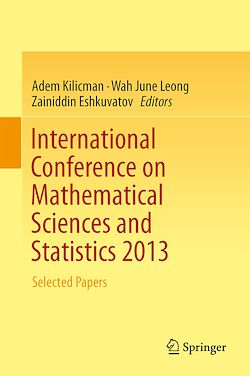# International Conference on Mathematical Sciences and Statistics 2013

-English
295 Pages

Description

This volume is devoted to the most recent discoveries in mathematics and statistics. It also serves as a platform for knowledge and information exchange between experts from industrial and academic sectors. The book covers a wide range of topics, including mathematical analyses, probability, statistics, algebra, geometry, mathematical physics, wave propagation, stochastic processes, ordinary and partial differential equations, boundary value problems, linear operators, cybernetics and number and functional theory. It is a valuable resource for pure and applied mathematicians, statisticians, engineers and scientists.

Subjects

##### Classwork

Informations